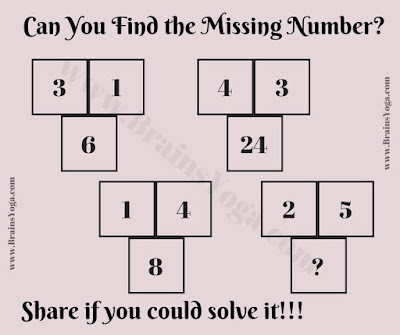Here is the Brain Teaser which will test your Logical Reasoning. Here the group of three numbers is given. These three number relates to each other Logically or Mathematically. Can you find this logical or Maths relationship among these number and then find the missing number which will replace the question mark?Can you find the missing number?